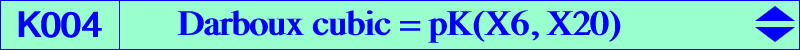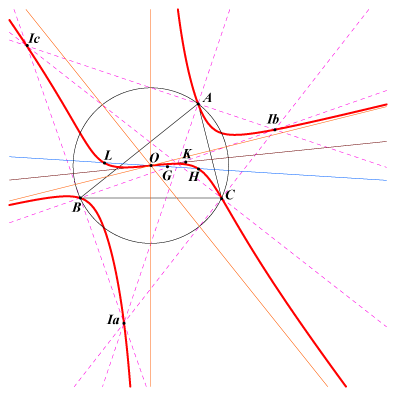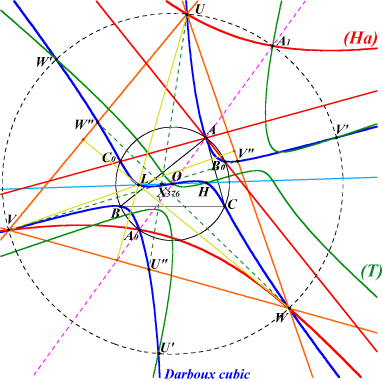X(1), X(3), X(4), X(20), X(40), X(64), X(84), X(1490), X(1498), X(2130), X(2131), X(3182), X(3183), X(3345), X(3346), X(3347), X(3348), X(3353), X(3354), X(3355), X(3472), X(3473), X(3637), X(40851), X(40852) antipodes of A, B, C on the circumcircle excenters, infinite points of the altitudes CPCC or H-cevian points, see Table 11, their reflections about X(3), their isogonal conjugates foci of the inconic with center X(3), X1-OAP points, see K033, K806, K807 and also Table 53 other points belowThe Darboux cubic is the isogonal pK with pivot the de Longchamps point L = X(20). See Table 27. It is the only isogonal central pK. Its center is O. Hence, it is a pK++. See also table 15. It is anharmonically equivalent to the Thomson cubic. See Table 21. K004 is a member of the class CL043 : it meets the circumcircle at A, B, C and at their antipodes where the tangents are concurrent at the point X(1498). See also Q063. K004 is a circumcevian pK. See CL072. The isotomic transform of K004 is K183. The symbolic substitution SS{a -> √a} transforms K004 into K202. K004 is its own sister as in Table 58. Other sisters are studied in CL065. Locus properties (see also Table 6) Locus of point P whose pedal (resp. antipedal) triangle is a cevian (resp. anticevian) triangle, the perspector being on the Lucas cubic (resp. Thomson cubic). For this reason, the Darboux cubic is called 1-pedal cubic in Pinkernell's paper. Locus of point P whose pedal and anticevian triangles are perspective, the perspector being on the Thomson cubic. Denote by PaPbPc the pedal triangle of point P and by T1, T2, T3, T4, T5, T6 the areas of triangles PBPa, PPaC, PCPb, PPbA, PAPc, PPcB respectively. The locus of point P such that T1*T3*T5 = T2*T4*T6 is the Darboux cubic. See also K003 (property 3) and Q082. The internal bisector at P of the triangle PPbPc meets PbPc at A1. B1 and C1 are defined similarly. The triangles ABC and A1B1C1 are perspective if and only if P lies on the Darboux cubic (Antreas Hatzipolakis, Hyacinthos #1945). The perpendicular at P to the line PA meets the lines AC, AB at Bc, Cb respectively. Define Ca, Ac, Ab, Ba similarly. The triangles AbBcCa and AcBaCb have equal areas if and only if P lies on the Darboux cubic (Paul Yiu, Hyacinthos #1959). Let P be a point with traces A_P, B_P, C_P. Denote by L1 the line joining the perpendicular feet of A_P on the cevians BB_P and CC_P. Let L2, L3 be the two lines analogously defined. The triangle bounded by L1, L2, L3 is perspective with ABC if and only if P lies on the Darboux cubic (together with the line at infinity, the three circles with diameters BC, CA, AB, and the Yiu quintic Q006) (Paul Yiu, Hyacinthos #1967). Jean-Pierre Ehrmann observes that these three lines are in fact concurrent if and only if P lies on Q006. See the related property 22. Denote by PaPbPc the pedal triangle of point P and by Qa, Qb, Qc the intersections of the lines AP and PbPc, BP and PcPa, CP and PaPb respectively. The triangles PaPbPc and QaQbQc are perspective if and only if P lies on the Darboux cubic (together with the line at infinity and the circumcircle). This question was raised by Nikolaos Dergiades and answered by Antreas Hatzipolakis (Hyacinthos #8336 & sq.) who asks two other questions answered at Q007 and Q009. Locus of point P such that the pedal and antipedal triangles of P are perspective (together with the line at infinity and the circumcircle). Locus of point P such that the six vertices of the pedal and cevian (or antipedal and anticevian) triangles of P lie on a same conic. See also Table 11. The locus of P whose anticevian or antipedal triangle is perspective to the hexyl triangle is K343. In the anticevian case, the locus of the perspector is the Darboux cubic. The locus of P whose cevian triangle is perspective to the hexyl triangle is K344. The locus of the perspector is the Darboux cubic. Locus of point P such that the pedal triangle of P and the anticevian triangle of the isogonal conjugate of P are perspective. Locus of point P such that the antipedal triangle of P and the cevian triangle of the isogonal conjugate of P are perspective. Denote by PaPbPc the pedal triangle of point P. The circles PAPa, PBPb, PCPc form a pencil if and if P lies on the Darboux cubic (together with the line at infinity) (Hyacinthos #6329 and related messages). In this case, they have two common (real) points P and P' which lies on Q071, the Darboux quintic. The lines joining the vertices of ABC with the midpoints of the sides of the pedal triangle of P concur at Q if and only if P is on the Darboux cubic. Q lies on the Thomson cubic. (Francisco Garcia Capitan, private message, 2009-01-04). See other related properties in K1092. Locus of P such that ABC and the pedal of the pedal of P are perspective (Nikolaos Dergiades, ADGEOM #132). See K645. Let (C) be any conic inscribed in ABC. Let P be a point with pedal triangle PaPbPc. The tangents (other than the sidelines of ABC) drawn from Pa, Pb, Pc to (C) form a triangle perspective to PaPbPc if and only if P lies on K004 (Angel Montesdeoca, private message, 2016-12-03). See K647 for another related locus property. The perpendiculars at A, B, C to the cevians of a point M meet the opposite sidelines of ABC at A', B', C' respectively. These points are collinear if and only if M lies on K004 (answers a question raised by Dominique Roux, 2018-10-10). See also here for other properties (Angel Montesdeoca, 2019-08-26, in Spanish) Let P be a point with cevian triangle PaPbPc. Denote by La the line joining the perpendicular feet of Pa on the lines AB, AC and let Lb, Lc be the two lines analogously defined. The triangle bounded by La, Lb, Lc is perspective with ABC if and only if P lies on the Darboux cubic, together with the circumcircle and a quartic which is the isogonal transform of the circum-conic of the tangential triangle with center O. This quartic is the locus of P such that La, Lb, Lc are concurent (Fancisco Garcia Capitan, private message 2020-03-26). See the related property 6. See K243. See Q172. See K099. Let P be a point with pedal triangle A'B'C'. Denote by La the radical axis of circles ABB' and ACC'. Define Lb, Lc cyclically. The lines La, Lb, Lc are concurrent (at Q) if and only if P lies on the Darboux cubic. The locus of Q is K002 (Ercole Suppa, private message, 2022-08-15). The perpendicular from P to BC intersects the sidelines AC, AB at Ab, Ac. The tangents to the circle (AAbAc) at Ab, Ac intersect at A1. Define B1, C1 cyclically. A, B, C, A1, B1, C1 lie on a same conic if and only if P lies on the Darboux cubic, together with the altitudes of ABC ((Kadir Altintas, private message, 2023-03-11. See also Euclid #5786).Other properties (Ha) is the hyperbola centered at A, passing through the antipode Ao of A in the circumcircle, through its reflection A1 in A and whose asymptotes are the perpendiculars at A to the sidelines AB and AC (these are the lines ABo and ACo). (Ha) meets the circle C(O,3R) at four points A1 and U, V, W. Naturally, U, V, W also lie on two similar hyperbolas (Hb) and (Hc) and the isogonal conjugates U*, V*, W* of U, V, W lie on K004. U, V, W and their reflections U', V', W' in O are six points on the Darboux cubic. Furthermore, the triangle UVW has orthocenter L, centroid X(376) (the reflection of G in O) and the midpoints U", V", W" also lie on K004. They are the isogonal conjugates of U', V', W' and the midpoints of LU', LV', LW'. Obviously, the reflections U3, V3, W3 of U", V", W" about X(3) also lie on K004.U', V', W', A1, B1, C1 are the six points where C(O,3R) meets (T), the homothetic of the Thomson cubic under h(O,3), thus they are the images of the vertices of the Thomson triangle under the same homothety. U', V', W' also lie on three rectangular hyperbolas (H'a), (H'b), (H'c) passing through H. (H'a) contains A, the reflection of L in A and its asymptotes are parallel to the bisectors at A. Its center is the homothetic of L under h(A,-1/2). Its tangent at A is the line AX(64) i.e. it is tangent at A to K004. Most of these points are directly related to the vertices Q1, Q2, Q3 of the Thomson triangle : U, U', U", U3 are the images of Q1 under h(X3, -3), h(X3, 3), h(X4, 3/2), h(X631, -3/2) respectively. The other points are defined similarly.A remarkable pencil of cubics The Darboux cubic K004 and the decomposed cubic which is the union of the circumcircle and the Euler line generate a pencil of central cubics with center O passing through H, X(20) and the reflections A', B', C' of A, B, C about O. Each cubic is spK(P, Q) in CL055 where P is a point on the Euler line and Q is the midpoint of PX(20). spK(P,Q) contains the infinite points of pK(X6, P) and the isogonal conjugate of P. This pencil also contains (apart K004 which is the only pK) the cubics K047, K080, K426, K443, K566 corresponding to K002, K003, pK(X6, X3146), K006, K005 respectively, and also K706, K814, K850, K918. Note that K426 and K443 are psK cubics. See Pseudo-Pivotal Cubics and Poristic Triangles. These cubics are those in the column P = [X20] of Table 54.K004 and the foci of the Steiner inellipse The Darboux cubic K004 is the homothetic of K758 under h(X3, 3). Since this latter cubic contains the four foci of the Steiner inellipse, K004 must contain their images under the same homothety. h(X4, 3) transforms these same foci into four other points, also on K004, which are the reflections about X(3) of the four previous points.Group structure and organization of points on the Darboux cubic The usual group structure is easily adapted on K004 : the sum M+N of two points is the isogonal conjugate of the third intersection S of K004 with the line MN and, obviously, X(20), S, M+N are collinear. The pivot X(20) is the neutral element 0 of the group. Following Fred Lang (Forum Geometricorum, vol.2, 2002, pp.135--146), we construct the following table (splitted into a negative part and a positive part) taking into account that K004 is stable under four transformations (detailed underneath) namely isogonal conjugation, reflection about X(3), X(20)-Ceva conjugation, X(64)-crossconjugation.n -8 -7 -6 -5 -4 -3 -2 -1 0 P X(3355) X(3472) X(2130) X(3353) X(3183) X(3182) X(1498) X(1490) X(20) Q X(3637) X(2131) X(3348) X(3346) X(64)0 1 2 3 4 5 6 7 8 9 10 11 12 13 14 X(20) X(40) X(3) X(1) X(4) X(84) X(64) X(3345) X(3346) X(3347) X(3348) X(3354) X(2131) X(3473) X(3637) X(64) X(4) X(3) X(20) X(1498) X(3183) X(2130) X(3355)Each point P on K004 corresponds to an integer n and Q = tg(P) denotes the tangential of P. Three points on K004 are collinear if and only if their sum is 6. This gives the folllowing assertions generalized below. • the sum of two isogonal conjugates P, P* is 6 since they are collinear with the neutral element X(20). • the sum of two points P, P' symmetric about X(3) is 4 since X(3) is 2. Equivalently, the arithmetic mean is 2. • the sum of P and its X(20)-Ceva conjugate X20/P is 0 since they are collinear with X(64), the tangential of X(20) with value 6. • the sum of P and its X(64)-cross conjugate X64©P is 12 since they are collinear with X(2130), the tangential of X(64) with value -6. The left-hand table shows a selection of points deduced from P with their corresponding integer. The right-hand table shows pairs of points with sum s hence collinear with a third point S of K004.P n P'* 2 + n P* 6 - n P*' -2 + n P' 4 - n tg(P*) -6 + 2n Q = tg(P) 6 - 2n Q* 2n X20/P - n tg(P') -2 + 2n X20/P* -6 + n Q' -2 + 2n X20/P' -4 + n X64©P 12 - n
 s S example / remark 0 X(64) X(20)-Ceva conjugates 1 X(84) X(1) and X(1498) 2 X(4) X(1) and X(1490) 3 X(1) X(3) and X(40) 4 X(3) symmetric about X(3) 5 X(40) X(1) and X(3) 6 X(20) isogonal conjugates 7 X(1490) X(1) and X(4) 8 X(1498) X(1) and X(84) 9 X(3182) X(1) and X(64) 12 X(2130) X(64)-cross conjugates

Remark : tg(P') = tg(P)' with same number -2 + 2n which is obvious geometrically.In other words, this gives a quick and easy method to find the third point of K004 on a line passing through two given points. For example, the third point P on X(3183)-X(3473) is given by -4+13+ n = 6 hence n = -3 so P = X(3182). Similarly, it is easy to identify the tangential of any point on K004.